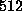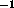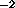Next: DETECT Up: DESCRIPTION OF OBSERVATION-LEVEL PROGRAMS Previous: CAST_PART_HRI

Purpose: To smooth the fullimages with an adaptive-filtering algorithm. For each unmasked pixel, the program will average neighboring pixels within a circle of increasing radius until a selected number of counts is reached. The original pixel is then given the average surface brightness for the pixels within the circle.

File Input:
1) Observation data set fits files - none
2) Other fixed input - after the band has been selected, the program reads in the appropriate range of cast count images (b_n.fits for PSPC observations, counts.fits for HRI observations), background images (back_n.fits for PSPC observations from the output of FINAL_IMAGE, back.fits for HRI observations) and exposure maps (expose_n.fits for PSPC observations, expose.fits for HRI observations).

Interactive Input:
1) Number of counts for the adaptive filter.
2) Scale factor for output - provides for a multiplicative scaling of the data.
3) Fractional limit for mask - ADAPT will find the average non-zero value for the mask and exclude all pixels where the mask value is less than the fractional limit multiplied by the average value. (Values of 0.02 for PSPC data and 0.2 for HRI data are reasonable.)
4) Binning factor - ADAPT will bin by 1, 2, or 4 pixels in each dimension before smoothing, as selected by 1, 2, and 4, respectively.
5) Band range to be smoothed - the range of bands to be smoothed for the image (i.e., ``2 2'' will smooth band R2, ``4 5'' will smooth band R45, and ``8 2'' will smooth band R1L2). An input of ``0 0'' will smooth HRI data.
Fits file adapt_n.fits where n is the band (e.g., adapt_5.fits) or band range (e.g., adapt_47.fits), of the smoothed image. The file contains the count rate in units of counts sarcmin. The HRI file is adapt.fits. Output files are I*2 and will saturate at 0.32 counts sarcminwith a scale factor of 1.0.